# High School Math : Transformations of Polynomial Functions

## Example Questions

### Example Question #1 : Transformations Of Polynomial Functions

List the transformations that have been enacted upon the following equation: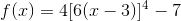vertical stretch by a factor of 4

horizontal compression by a factor of 6

vertical translation 7 units down

horizontal translation 3 units left

vertical compression by a factor of 4

horizontal stretch by a factor of 6

vertical translation 7 units down

horizontal translation 3 units right

vertical stretch by a factor of 4

horizontal compression by a factor of 6

vertical translation 7 units down

horizontal translation 3 units right

vertical stretch by a factor of 1/4

horizontal compression by a factor of 1/6

vertical translation 7 units down

horizontal translation 3 units right

vertical stretch by a factor of 4

horizontal stretch by a factor of 6

vertical translation 7 units down

horizontal translation 3 units right

vertical stretch by a factor of 4

horizontal compression by a factor of 6

vertical translation 7 units down

horizontal translation 3 units right

Explanation:

Since the equation given in the question is based off of the parent function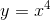, we can write the general form for transformations like this: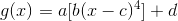determines the vertical stretch or compression factor.

• If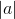is greater than 1, the function has been vertically stretched (expanded) by a factor of.
• Ifis between 0 and 1, the function has been vertically compressed by a factor of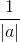.

In this case,is 4, so the function has been vertically stretched by a factor of 4.determines the horizontal stretch or compression factor.

• If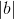is greater than 1, the function has been horizontally compressed by a factor of.
• Ifis between 0 and 1, the function has been horizontally stretched (expanded) by a factor of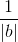.

In this case,is 6, so the function has been horizontally compressed by a factor of 6. (Remember that horizontal stretch and compression are opposite of vertical stretch and compression!)determines the horizontal translation.

• Ifis positive, the function was translatedunits right.
• Ifis negative, the function was translatedunits left.

In this case,is 3, so the function was translated 3 units right.determines the vertical translation.

• Ifis positive, the function was translatedunits up.
• Ifis negative, the function was translatedunits down.

In this case,is -7, so the function was translated 7 units down.